# Handwriting Generaton with Recurrent Neural Networks and Mixture of Gaussians¶

Thomas Viehmann [email protected]

This is a PyTorch implementation of the handwriting generation in Alex Graves' classic paper Generating Sequences with Recurrent Neural Networks. In addition to replicating the model, I wanted to explore recreating the visualisations that Graves uses.

I also studied Alexandre de Brébisson's Theano implementation, but all errors are my own.

I can highly recommend the original paper, I enjoyed reading it a lot.

Note: This notebook is written using PyTorch 0.4 (master at the time of writing).

At popular request, I have you can get pretrained model weights. Use them with the load_from_hdf5 code below. They are not particularly tweaked but just the ones that I got when I ran the notebook for the outputs you see below.

## Overview¶

Besically we have the following architecture (shown in the diagram):

• The triplets of relative movements in $x$ and $y$ direction and $pen$ (note this is different to Graves' notation, who uses $x$ for the triple) and a (attention-) mixture $w$ of one-hot encoded characters are fed into an LSTM, producing a hidden state $h$.
• The Attention takes the sequence of one-hot encoded characters ($c$ in Graves) and the hidden state $h$ to weight $c$ and give a new mixture $w$ of $c$ items. The attention component remembers the location of the attention modes.
• A Mixture Density Network (see a short notebook here) takes the LSTM output $h$ and the attention-modulated character information $w$ to compute a Gaussian density mixture for the coordinates and a Bernoulli parameter for pen up/pen down. In training the negative log likelihood of the actual movement and pen is used, and for prediction, movement and pen are sampled.
• A module wraps the three components and steps through the sequence.

(I honestly don't know how to make those esges between timesteps closer...)

In :
import graphviz
g = graphviz.Digraph(graph_attr=dict(size="12,12"))
with g.subgraph() as dot:
g.node("pts(t-1)",label="(X,Y,Pen)(t-1)", shape="ellipse", style="filled")
g.node("txt",label="Text", style="filled")
g.node("w(t-1)", style="filled")
g.edge("pts(t-1)","txt", style="invis")
g.edge("txt","w(t-1)", style="invis")
for i,timestep in enumerate(["t","t+1", "t+2"]):
with g.subgraph(name="cluster_{}".format(timestep)) as dot:
dot.attr(label='Step {}'.format(timestep))
dot.attr(sortv=str(i+1))
dot.node("LSTM{}".format(timestep),label="LSTM", shape="box", style="rounded")
dot.node("GMM{}".format(timestep),label="Mixture Density", shape="box", style="rounded")
dot.node("ATT{}".format(timestep),label="Attention", shape="box", style="rounded")
dot.node("pts({})".format(timestep),label="(X,Y,Pen)({})".format(timestep), shape="ellipse", style="filled")
dot.node("h({})".format(timestep), shape="ellipse", style="filled")
dot.node("w({})".format(timestep), shape="ellipse", style="filled")
dot.edge("h({})".format(timestep), "ATT{}".format(timestep))
dot.edge("LSTM{}".format(timestep),"h({})".format(timestep))
dot.edge("h({})".format(timestep), "GMM{}".format(timestep))
dot.edge("ATT{}".format(timestep), "w({})".format(timestep))
dot.edge("w({})".format(timestep), "GMM{}".format(timestep))
dot.edge("GMM{}".format(timestep), "pts({})".format(timestep), style="dashed", label="predict")

g.edge("pts(t-1)", "LSTMt", constraint="false")
g.edge("w(t-1)", "LSTMt", constraint="false")
g.edge("txt", "ATTt", constraint="false")
g.edge("txt", "ATTt+1", constraint="false")
g.edge("txt", "ATTt+2", constraint="false")
g.edge("w(t)","LSTMt+1",constraint="false")
g.edge("w(t+1)","LSTMt+2",constraint="false")
g.edge("pts(t)","LSTMt+1",constraint="false")
g.edge("pts(t+1)","LSTMt+2",constraint="false")
g

Out:

First let us import something.

In :
import os
import sys
import pickle
import time
import collections
import numpy
import IPython
import cairocffi as cairo
from matplotlib import pyplot
import matplotlib
import torchtext
import tqdm
import glob
import lxml.etree
%matplotlib inline
import torch
torch.manual_seed(42)

Out:
<torch._C.Generator at 0x7f8e0ec5f650>

## The dataset¶

The set is split into a training and three test sets, the latter being labeled v, f, and t. Following Graves, Section 4, we use the v set as validation and combine the other three for training.

I used to have a separate preprocessing notebook, but preprocessing takes less than 30 seconds for me, so I just moved it here...

In :
iam_dir = "/home/datasets/iam-online-handwriting-database/"
orig_trainset = [l.strip() for l in open(os.path.join(iam_dir, "trainset.txt"))]
orig_testset_v = [l.strip() for l in open(os.path.join(iam_dir, "testset_v.txt"))] # smallest
orig_testset_f = [l.strip() for l in open(os.path.join(iam_dir, "testset_f.txt"))]
orig_testset_t = [l.strip() for l in open(os.path.join(iam_dir, "testset_t.txt"))]

trainset = orig_trainset + orig_testset_f + orig_testset_t
validset = orig_testset_v


The dataset contains ascii files with the text and xml-based representations of the strokes.

In :
blacklist = {"a08-551z-08","a08-551z-09"} # these seem broken

def process_set(theset):
txts = {}
for i,n in enumerate(theset):
txtfn = "/home/datasets/iam-online-handwriting-database/ascii/{}/{}/{}.txt".format(n[:3], n[:7], n)
for j,t in enumerate(open(txtfn).read().split("CSR:").strip().split("\n")):
txts['{}-{:02d}'.format(n,j+1)] = t
samples = {}
for i,n in tqdm.tqdm(enumerate(theset),total=len(theset)):
globmask = "/home/datasets/iam-online-handwriting-database/lineStrokes/{}/{}/{}-*.xml".format(n[:3], n[:7], n)
for fn in glob.glob(globmask):
key = (os.path.splitext(os.path.basename(fn)))
if key not in blacklist:
root = lxml.etree.parse(fn).getroot()
strokes_list = []
for s in root.find("StrokeSet").findall("Stroke"):
pts = torch.FloatTensor([(float(p.attrib['x']), float(p.attrib['y']),0.0) for p in s.findall("Point")])
pts[-1,-1] = 1
strokes_list.append(pts)
strokes = torch.cat(strokes_list, dim=0)
(min_x,min_y),_ = torch.min(strokes[:,:2],dim=0)
(max_x,max_y),_ = torch.max(strokes[:,:2],dim=0)
rel_strokes = torch.cat([torch.zeros(1,3),
torch.cat([strokes[1:,:2]-strokes[:-1,:2],strokes[1:,2:]],dim=1)], dim=0)
if rel_strokes.abs().max()<1000: # we assume that such large moves are broken
samples[key] = {"strokes":strokes_list, "minmax":(min_x,min_y,max_x,max_y), "rel_strokes":rel_strokes,
"txt": txts[key]}
return samples

In :
training_samples = process_set(trainset)
#torch.save(training_samples, "preprocessed-training.pt")
val_samples = process_set(validset)
#torch.save(val_samples, "preprocessed-validation.pt")

100%|██████████| 1535/1535 [00:17<00:00, 88.31it/s]
100%|██████████| 192/192 [00:02<00:00, 83.38it/s]

In :
x = torch.cat([d["rel_strokes"] for d in training_samples.values()], dim=0)
mean,std = x[:,:2].mean(0),x[:,:2].std(0)
print ("mean", mean, "std", std)

mean
8.1842
0.1145
[torch.FloatTensor of size (2,)]
std
40.3660
37.0441
[torch.FloatTensor of size (2,)]



We use a torchtext dataset to use our preprocessed IAM online handwriting data.

In :
char_dict = {k:v for v,k in enumerate(' !"#%&\'()+,-./0123456789:;?ABCDEFGHIJKLMNOPQRSTUVWXYZ[]abcdefghijklmnopqrstuvwxyz')}
inv_char_dict = {v:k for k,v in char_dict.items()}
n_chars = len(char_dict)

class DS(torchtext.data.Dataset):
def __init__(self, samples, mean, std):
self.myfields = [('txt',torchtext.data.Field(use_vocab=True,tokenize=list, eos_token='<eos>')),
('txtn',torchtext.data.Field(use_vocab=False,pad_token=0)),
('txtlen',torchtext.data.Field(use_vocab=False)),
('txtmask',torchtext.data.Field(use_vocab=False,pad_token=0, tensor_type=torch.FloatTensor)),
('xs',torchtext.data.Field(use_vocab=False, pad_token=-1, tensor_type=torch.FloatTensor)),
('ys',torchtext.data.Field(use_vocab=False, pad_token=-1, tensor_type=torch.FloatTensor)),
('pen',torchtext.data.Field(use_vocab=False,pad_token=-1)),
('ptslen',torchtext.data.Field(use_vocab=False))
]
self.coord_mean = mean
self.coord_std  = std
examples = []
for s in samples.values():
txt = [c for c in s["txt"] if c in char_dict]
txtn = torch.LongTensor([char_dict[i] for i in txt])
txtlen = torch.LongTensor([len(txt)])
txtmask = torch.ones(len(txt))
stroke = s['rel_strokes']
xs = stroke[:,0]
ys = stroke[:,1]
pen = stroke[:,2]
ptslen = torch.LongTensor([len(pen)])
if xs.abs().max()<1000 and ys.abs().max()<1000 and len(txt)>=20:
xs = (xs-self.coord_mean)/self.coord_std
ys = (ys-self.coord_mean)/self.coord_std
examples.append(torchtext.data.Example.fromlist([txt, txtn, txtlen, txtmask,
xs, ys, pen, ptslen
], self.myfields))
super(DS, self).__init__(examples, self.myfields)
self.myfields.build_vocab(self)
def sort_key(self, ex):
return len(ex.txt)
def tensor_to_str(self,t):
return ''.join([self.fields['txt'].vocab.itos[c] for c in t if c >= 3])
def tensor_to_str2(self,t):
return ''.join([inv_char_dict[c] for c in t])

train_ds = DS(training_samples, mean, std)
val_ds   = DS(val_samples, mean, std)


Here is both a matplotlib-based and a cairo-based function to display a stroke. The cairo-based function is a lot faster and optionally add random shear and rotation, the matplotlib-based one offers color.

In :
def show_stroke(x, colors=None):
x= x[:(torch.arange(0,x.size(0))[x[:,2]>-0.0001].size(0))] # only used bits
stroke = (x[:,:2]*train_ds.coord_std.unsqueeze(0)+train_ds.coord_mean.unsqueeze(0)).cumsum(0)
stroke[:,1] *= -1
pen = x[:,2]
xmin,ymin = stroke.min(0)
xmax,ymax = stroke.max(0)

actions = [matplotlib.path.Path.MOVETO]
coords = []
for c,p in zip(stroke, pen):
if p >=-0.0001:
if p==1 or len(actions)==0:
actions.append(matplotlib.path.Path.MOVETO)
else:
actions.append(matplotlib.path.Path.LINETO)
coords.append((c,c))
actions = actions[:-1]
ax = pyplot.gca()
ax.set_xlim(xmin, xmax)
ax.set_ylim(ymin, ymax)

if colors is None:
path = matplotlib.path.Path(coords, actions)
patch = matplotlib.patches.PathPatch(path, facecolor='none')
ax.add_patch(patch)
else:
pos = coords
curpos = pos
for pos,a,col in zip(coords, actions, colors):
if a == matplotlib.path.Path.LINETO:
ax.add_line(matplotlib.lines.Line2D((curpos,pos), (curpos,pos), axes=ax, color=col))
curpos = pos

def stroke_to_image(x, target_size = (1280,64), randomize=False):
stroke = (x[:,:2]*train_ds.coord_std.unsqueeze(0)+train_ds.coord_mean.unsqueeze(0)).cumsum(0)
pen = x[:,2]
if randomize:
shear_prob = 0.5
shear_prec = 4.0
if torch.rand(1) > shear_prob:
shear_sigma = 1/shear_prec**0.5
shear_theta = 0.5*torch.randn(1)
else:
shear_theta = torch.zeros(1)
rot_prob = 0.5
rot_prec = 4.0
if torch.rand(1) > rot_prob:
rot_sigma = 1/rot_prec**0.5
rot_theta = 0.5*torch.randn(1)
else:
rot_theta = torch.zeros(1)

(min_x,min_y),_ = torch.min(stroke[:,:2],dim=0)
(max_x,max_y),_ = torch.max(stroke[:,:2],dim=0)
stroke[:,0] -= min_x
stroke[:,1] -= min_y
min_x, min_y = 0.0,0.0
max_x, max_y = max_x-min_x, max_y-min_y

stroke[:,0] += stroke[:,1]*torch.sin(shear_theta)

stroke[:,0] = stroke[:,0]*torch.cos(rot_theta)+stroke[:,1]*torch.sin(rot_theta)
stroke[:,1] = stroke[:,1]*torch.cos(rot_theta)-stroke[:,0]*torch.sin(rot_theta)

(min_x,min_y),_ = torch.min(stroke[:,:2],dim=0)
(max_x,max_y),_ = torch.max(stroke[:,:2],dim=0)
stroke[:,0] -= min_x
stroke[:,1] -= min_y
min_x, min_y = 0.0,0.0
max_x, max_y = max_x-min_x, max_y-min_y

factor = min(target_size/max(max_x-min_x,0.001),target_size/max(max_y-min_y,0.001),1)
xmin,ymin = stroke.min(0)
xmax,ymax = stroke.max(0)

imwidth, imheight = int(xmax*factor)+2, int(ymax*factor)+2
surface = cairo.ImageSurface (cairo.FORMAT_A8, imwidth, imheight)
ctx = cairo.Context(surface)

ctx.scale (factor, factor) # Normalizing the canvas
ctx.rectangle (0, 0, xmax+5/factor, ymax+5/factor) # Rectangle(x0, y0, x1, y1)
ctx.set_source_rgba (0.0, 0.0, 0.0, 0.0) # Solid color
ctx.fill ()
next_action = 1
coords = []
for c,p in zip(stroke, pen):
if p >=-0.0001:
if next_action:
ctx.move_to(c+1,c+1)
else:
ctx.line_to(c+1,c+1)
next_action = p>0.5
ctx.set_source_rgba(1.0, 1.0, 1.0, 1.0) # Solid color

if randomize:
linewidth = (1+torch.rand(1))/factor
else:
linewidth = 2/factor
ctx.set_line_width (linewidth)
ctx.stroke ()

buf = surface.get_data()
data = numpy.ndarray(shape=(imheight, (imwidth+3)//4*4),#(WIDTH+7)//8),
dtype=numpy.uint8,
buffer=buf)[:,:imwidth]
data = 1-(data>0)
data = torch.FloatTensor(data)
return data


Let us see an example of our data:

In :
b = train_ds
pts = torch.stack([b.xs,b.ys,b.pen.float()],dim=-1)
pyplot.figure(figsize=(10,5))
pyplot.imshow(stroke_to_image(pts[:b.ptslen].cpu()), cmap=pyplot.cm.gray)
''.join(b.txt)

Out:
'at Lancaster House despite the crisis which'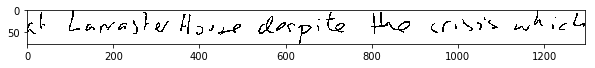## The network¶

### Mixture Density Network¶

The mixture density network, described in Graves Section 4.1, consists of a linear layer and parameter transforms in compute_params. The parameters are

• a vector $\pi$ with the weights of the mixture components,
• vectors (one element per mixture component)mean_x, mean_y ($\mu$ in Graves), std_x, std_y ($\sigma$), and $\rho$,
• a probaility bernoulli ($e$ in Graves) of the pen being up (i.e. $1$ means no pen).

Then for training (in forward), the negative log likelihood is computer. For prediction (predict), movement and pen indicator are sampled. In the prediction, we allow for bias ($b$) as in Graves Section 5.4.

We found that the training sometimes runs into NaN unless we use some regularisation, we do so via a parameter eps. This may be due to us using single precision floating point numbers rather than double precision or a too aggressive learning rate. We also see below that we do not seem to achieve the deterministic outputs when the bias is high.

In :
class MixtureGaussians2DandPen(torch.nn.Module):
"""Gaussian mixture module as in Graves Section 4.1"""
def __init__(self, n_inputs, n_mixture_components, eps=1e-6):
# n_inputs = dimension N of h^n_t in Graves eq. (17)
# n_mixture_components = number M of mixture components in Graves eq. (16)
super().__init__()
self.n_mixture_components = n_mixture_components
self.eps = eps
# linear layer as in Graves eq. (17)
# ((proportions, m_x, m_y, s_x, s_y, rho)*n_mixture_components, pen)
self.linear = torch.nn.Linear(n_inputs, n_mixture_components *6 + 1)

def compute_parameters(self, h, bias):
# h: batch x n_inputs
# The output of the input layer is batch x ...
y_hat = self.linear(h)
if y_hat.requires_grad:
y_hat.register_hook(lambda x: x.clamp(min=-100, max=100))

M = self.n_mixture_components
# note that our ordering within y_hat is different to Graves eq (17)
# we also incorporate the bias b given in Graves eq (61) and (62)
# we have a regularisation self.eps that Graves does not have in the paper
pi = torch.nn.functional.softmax(y_hat[:, :M]*(1 + bias),1) # Graves eq (19)
mean_x = y_hat[:, M:M*2]                                      # Graves eq (20)
mean_y = y_hat[:, M*2:M*3]
std_x = torch.exp(y_hat[:, M*3:M*4] - bias) + self.eps        # Graves eq (21)
std_y = torch.exp(y_hat[:, M*4:M*5] - bias) + self.eps
rho = torch.tanh(y_hat[:, M*5:M*6])                           # Graves eq (22)
rho = rho/(1+self.eps)
bernoulli = torch.sigmoid(y_hat[:, -1])                       # Graves eq (18)
bernoulli = (bernoulli + self.eps) / (1 + 2*self.eps)
#bernoulli = 1/(1+torch.exp(-(1+bias)*((torch.log(bernoulli)-torch.log(1-bernoulli))+3*(1-torch.exp(-bias))))) # this is NOT covered by Graves: Bias in the Bernoulli
return pi, mean_x, mean_y, std_x, std_y, rho, bernoulli

def predict(self, h, bias=0.0):
pi, mean_x, mean_y, std_x, std_y, rho, bernoulli = self.compute_parameters(h, bias)

mode = torch.multinomial(pi.data,1) # choose one mixture component

m_x = mean_x.gather(1, mode).squeeze(1) # data for the chosen mixture component
m_y = mean_y.gather(1, mode).squeeze(1)
s_x = std_x.gather(1, mode).squeeze(1)
s_y = std_y.gather(1, mode).squeeze(1)
r   = rho.gather(1, mode).squeeze(1)

normal = rho.new().resize_((h.size(0), 2)).normal_()
x = normal[:, 0]
y = normal[:, 1]

x_n = (m_x + s_x * x).unsqueeze(-1)
y_n = (m_y + s_y * (x * r + y * (1.-r**2)**0.5)).unsqueeze(-1)

uniform = bernoulli.data.new(h.size(0)).uniform_()
pen = torch.autograd.Variable((bernoulli.data > uniform).float().unsqueeze(-1))

return torch.cat([x_n, y_n, pen], dim=1)

def forward(self, h_seq, mask_seq, tg_seq, hidden_dict=None):
# h_seq: (seq, batch, features),  mask_seq: (seq, batch), tg_seq: (seq, batch, features=3)
batch_size = h_seq.size(1)
h_seq = h_seq.view(-1, h_seq.size(-1))
tg_seq = tg_seq.view(-1, tg_seq.size(-1))
mask_seq = mask_seq.view(-1,)

atensor = next(self.parameters())
bias = torch.zeros((),device=atensor.get_device(), dtype=atensor.dtype)
pi, mean_x, mean_y, std_x, std_y, rho, bernoulli = self.compute_parameters(h_seq, bias)

if hidden_dict is not None:
hidden_dict["pi"].append(pi.data.cpu())
hidden_dict["mean_x"].append(mean_x.data.cpu())
hidden_dict["mean_y"].append(mean_y.data.cpu())
hidden_dict["std_x"].append(std_x.data.cpu())
hidden_dict["std_y"].append(std_y.data.cpu())
hidden_dict["rho"].append(rho.data.cpu())
hidden_dict["bernoulli"].append(bernoulli.data.cpu())

tg_x = tg_seq[:, 0:1]
tg_y = tg_seq[:, 1:2]
tg_pen = tg_seq[:, 2]

tg_x_s = (tg_x - mean_x) / std_x
tg_y_s = (tg_y - mean_y) / std_y

z = tg_x_s**2 + tg_y_s**2 - 2*rho*tg_x_s*tg_y_s # Graves eq (25)

if hidden_dict is not None:
hidden_dict["z"].append(z.data.cpu())

tmp = 1-rho**2
# tmp is ln (pi N(x, mu, sigma, rho)) with N as in Graves eq (24) (this is later used for eq (26))
mixture_part_loglikelihood = (-z / (2 * tmp)
-numpy.log(2*numpy.pi) - torch.log(std_x) - torch.log(std_y) - 0.5*torch.log(tmp)
+torch.log(pi))

# logsumexp over the mixture components
# mixture_log_likelihood the log in the first part of Graves eq (26)
mpl_max,_ = mixture_part_loglikelihood.max(1, keepdim=True)
mixture_log_likelihood = (mixture_part_loglikelihood-mpl_max).exp().sum(1).log()+mpl_max.squeeze(1)

# these are the summands in Graves eq (26)
loss_per_timestep = (-mixture_log_likelihood - tg_pen * torch.log(bernoulli) - (1-tg_pen) * torch.log(1 - bernoulli))

if hidden_dict is not None:
hidden_dict["loss_per_timestep"].append(loss_per_timestep.data.cpu())
# loss as in Graves eq (26)
loss = torch.sum(loss_per_timestep * mask_seq)/batch_size
return loss


### Attention Mechanism¶

Graves Section 5.1 describes a straightforward attention mechanism using a mixture-type weighting scheme and with moving modes. In addition to the one-hot encoded character sequence, the input for the attention mechanism is the LSTM-output $h$ and the previous time-steps mode locations $\kappa_{t-1}^k$. More precisely, weights $\phi$ are computed as $$\phi(t, u) = \sum_k \alpha_t^k \exp(-\beta_t^k (\kappa_t^k-u)^2)$$ with $t$ being the recurrence time step, $u$ the character location and $k$ is used to sum over the components. Here, $\alpha_t^k, \beta_t^k$ and $\kappa_t^k-\kappa_{t-1}^k$ are positive - mapped by $\exp$ from the output of a linear layer. For the $\kappa_t^k$, the difference is modelled to achieve better stationarity. The module returns the weight vector $\phi$ and the mode location vector $\kappa$.

Graves notes he does not normalize the weights.

In :
class Attention(torch.nn.Module):
def __init__(self, n_inputs, n_mixture_components):
super().__init__()
self.n_mixture_components = n_mixture_components
# linear layer from Graves eq (28)
self.linear = torch.nn.Linear(n_inputs, n_mixture_components*3)
def forward(self, h_1, kappa_prev, c_seq_len):
# h_1: batch x n_inputs, kappa_prev batch x n_mixture_components
K = self.n_mixture_components
params = torch.exp(self.linear(h_1)) # exp of Graves eq (48)
alpha = params[:,:K]                 # Graves eq (49)
beta  = params[:,K:2*K]              # Graves eq (50)
kappa = kappa_prev + 0.1*params[:,2*K:]  # Graves eq (51)
u = torch.arange(0,c_seq_len, out=kappa.new()).view(-1,1,1)
phi = torch.sum(alpha * torch.exp(-beta*(kappa-u)**2), dim=-1)
return phi, kappa


### LSTM¶

In order to be able to trade the LSTM cell for a GRU, I have a thin wraper around the LSTMCell module.

In :
class RNNCell(torch.nn.Module):
def __init__(self, input_size, hidden_size, bias=True):
super().__init__()
self.input_size = input_size
self.hidden_size = hidden_size
self.cell = torch.nn.LSTMCell(input_size, hidden_size, bias)
def forward(self, inp, hidden = None):
if hidden is None:
batch_size = inp.size(0)
hx = inp.new(batch_size, self.hidden_size).zero_()
cx = inp.new(batch_size, self.hidden_size).zero_()
hidden = (hx, cx)
return self.cell(inp, hidden)
def get_hidden(self, hidden):
return hidden


### Main Module¶

Tying things together is the main module. It is disappointingly heavyweight given that it mostly does administraton.

In :
class HandwritingModel(torch.nn.Module):
def __init__(self, n_hidden, n_chars, n_attention_components, n_gaussians, grad_clipping=10):
super(HandwritingModel, self).__init__()
self.n_hidden = n_hidden
self.n_chars = n_chars
self.n_attention_components = n_attention_components
self.n_gaussians = n_gaussians

self.attention = Attention(n_hidden, n_attention_components)
self.rnn_cell = RNNCell(3+self.n_chars, n_hidden)
self.grad_clipping = grad_clipping
self.mixture = MixtureGaussians2DandPen(n_hidden+self.n_chars, n_gaussians)

def rnn_step(self, inputs, h_state_pre, k_pre, w_pre, c, c_mask, mask=None, hidden_dict=None):
# inputs: (batch_size, n_in + n_in_c)
inputs = torch.cat([inputs, w_pre], dim=1)

# h: (batch_size, n_hidden)
h_state = self.rnn_cell(inputs, h_state_pre)
h = self.rnn_cell.get_hidden(h_state)
if h.requires_grad:
h.register_hook(lambda x: x.clamp(min=-self.grad_clipping, max=self.grad_clipping))

# update attention
phi, k =  self.attention(h, k_pre, c.size(0))
phi = phi * c_mask
# w: (batch_size, n_chars)
w = torch.sum(phi.unsqueeze(-1) * c, dim=0)
if mask is not None:
k = mask.unsqueeze(1)*k + (1-mask.unsqueeze(1))*k_pre
w = mask.unsqueeze(1)*w + (1-mask.unsqueeze(1))*w_pre
if w.requires_grad:
w.register_hook(lambda x: x.clamp(min=-100, max=100))
return h_state, k, phi, w

def forward(self, seq_pt, seq_mask, seq_pt_target, c, c_mask,
h_ini=None, k_ini=None, w_ini=None, hidden_dict=None):
batch_size = seq_pt.size(1)
atensor = next(m.parameters())

#if h_ini is None:
#    h_ini = self.mixture.linear.weight.data.new(batch_size, self.n_hidden).zero_()
if k_ini is None:
k_ini = atensor.new(batch_size, self.n_attention_components).zero_()
if w_ini is None:
w_ini = atensor.new(batch_size, self.n_chars).zero_()

# Convert the integers representing chars into one-hot encodings
# seq_str will have shape (seq_length, batch_size, n_chars)

c_idx = c
c = c.data.new(c.size(0), c.size(1), self.n_chars).float().zero_()
c.scatter_(2, c_idx.view(c.size(0), c.size(1), 1), 1)

seq_h = []
seq_k = []
seq_w = []
seq_phi = []
h_state, k, w = h_ini, k_ini, w_ini
for inputs, mask in zip(seq_pt, seq_mask):
h_state, k, phi, w = self.rnn_step(inputs, h_state, k, w, c, c_mask, mask=mask, hidden_dict=hidden_dict)
h = self.rnn_cell.get_hidden(h_state)
seq_h.append(h)
seq_k.append(k)
seq_w.append(w)
if hidden_dict is not None:
seq_phi.append(phi)
seq_h = torch.stack(seq_h,0)
seq_k = torch.stack(seq_k,0)
seq_w = torch.stack(seq_w,0)
if hidden_dict is not None:
hidden_dict['seq_h'].append(seq_h.data.cpu())
hidden_dict['seq_k'].append(seq_k.data.cpu())
hidden_dict['seq_w'].append(seq_w.data.cpu())
hidden_dict['seq_phi'].append(torch.stack(seq_phi,0).data.cpu())
seq_hw = torch.cat([seq_h, seq_w], dim=-1)

loss = self.mixture(seq_hw, seq_mask, seq_pt_target, hidden_dict=hidden_dict)
return loss

def predict(self, pt_ini, seq_str, seq_str_mask,
h_ini=None, k_ini=None, w_ini=None, bias=.0, n_steps=10000, hidden_dict=None):
# pt_ini: (batch_size, 3), seq_str: (length_str_seq, batch_size), seq_str_mask: (length_str_seq, batch_size)
# h_ini: (batch_size, n_hidden), k_ini: (batch_size, n_mixture_attention), w_ini: (batch_size, n_chars)
# bias: float    The bias that controls the variance of the generation
# n_steps: int   The maximal number of generation steps.
atensor = next(m.parameters())
bias = bias*torch.ones((),device=atensor.get_device(), dtype=atensor.dtype)
batch_size = pt_ini.size(0)
if k_ini is None:
k_ini = atensor.new(batch_size, self.n_attention_components).zero_()
if w_ini is None:
w_ini = atensor.new(batch_size, self.n_chars).zero_()

# Convert the integers representing chars into one-hot encodings
# seq_str will have shape (seq_length, batch_size, n_chars)

input_seq_str = seq_str
seq_str = pt_ini.data.new(input_seq_str.size(0), input_seq_str.size(1), self.n_chars).float().zero_()
seq_str.scatter_(2, input_seq_str.data.view(seq_str.size(0), seq_str.size(1) ,1), 1)
seq_str = torch.autograd.Variable(seq_str)

mask = torch.autograd.Variable(self.mixture.linear.weight.data.new(batch_size).fill_(1))
seq_pt = [pt_ini]
seq_mask = [mask]

last_char = seq_str_mask.long().sum(0)-1

pt, h_state, k, w = pt_ini, h_ini, k_ini, w_ini
for i in range(n_steps):
h_state, k, phi, w = self.rnn_step(pt, h_state, k, w, seq_str, seq_str_mask, mask=mask, hidden_dict=hidden_dict)
h = self.rnn_cell.get_hidden(h_state)
hw = torch.cat([h, w], dim=-1)
pt = self.mixture.predict(hw, bias)
seq_pt.append(pt)

last_phi = torch.gather(phi, 0, last_char.unsqueeze(0)).squeeze(0)
max_phi,_ = phi.max(0)
mask = mask * (1-(last_phi >= 0.95*max_phi).float())
seq_mask.append(mask)
if mask.data.sum()==0:
break
seq_pt   = torch.stack(seq_pt,0)
seq_mask = torch.stack(seq_mask,0)
return seq_pt, seq_mask


## Training¶

In :
n_hidden = 900
n_mixt_attention = 10
n_mixt_output = 20
batch_size = 50

train_it, = torchtext.data.BucketIterator.splits((train_ds,), batch_size=batch_size, repeat=False)
val_it,   = torchtext.data.BucketIterator.splits((val_ds,),   batch_size=batch_size, repeat=False)

m = HandwritingModel(n_hidden, n_chars, n_mixt_attention, n_mixt_output)
m.cuda()
# These are based on Graves Section 4.2.
opt = torch.optim.RMSprop(m.parameters(), lr=1e-4, eps=1e-4, alpha=0.95, momentum=0.9, centered=True)
losses = []


Now the training. If you want to skip the training, skip the next cell and enable the loading below.

In :
for epoch in range(50):
lasttime = time.time()
lastbatch = -1
epochstart = lasttime
for batch,i in enumerate(train_it):
#i_seq_pt, i_seq_tg, i_seq_mask, i_seq_str, i_seq_str_mask = [torch.autograd.Variable(torch.from_numpy(i).cuda()) for i in inputs]
#i_seq_str = i_seq_str.long()
i_seq_pt = torch.stack([i.xs, i.ys, i.pen.float()], dim=2)
i_seq_tg = i_seq_pt[1:]
i_seq_pt = i_seq_pt[:-1]
i_seq_mask = (i.pen[:-1]>=0).float()
i_seq_str = i.txtn
i_seq_str_mask = i.txtmask

opt.zero_grad()
res = m(i_seq_pt, i_seq_mask, i_seq_tg, i_seq_str, i_seq_str_mask)
res.backward()
losses.append(res.data)
if batch % 10 == 0:
losses = [float(l) for l in losses]
IPython.display.clear_output(wait=True)
pyplot.figure(figsize=(15,5))
pyplot.subplot(1,2,1)
pyplot.plot(losses)
if len(losses)>=50:
smoothed = (numpy.convolve(losses,numpy.ones(50)/50,'valid'))
pyplot.plot(numpy.arange(len(losses)-len(smoothed),len(losses)),smoothed)
print ("smoothloss", smoothed[-1])
pyplot.subplot(1,2,2)
with torch.no_grad():
i_seq_str = torch.autograd.Variable(torch.LongTensor([int(char_dict[c]) for c in "Die Katzen von Kopenhagen. "]).view(-1,1).cuda())
i_seq_str_mask = torch.autograd.Variable(torch.ones(i_seq_str.size()).cuda())
seq_pt, seq_mask = m.predict(i_seq_pt[0,:1], i_seq_str, i_seq_str_mask, bias=0.8)
lengths = seq_mask.sum(0).data.long()
idx = 0
show_stroke(seq_pt.data.cpu()[:lengths[idx],idx])
#''.join([inv_char_dict[c] for c in i_seq_str.data.cpu()[:,idx]])
pyplot.show()
print ("time per minibatch",(time.time()-lasttime)/(batch-lastbatch))
lastbatch = batch
lasttime = time.time()
print (epoch,'/', batch,'(of 208) :', res.item())

torch.nn.utils.clip_grad_norm(m.parameters(),5)
opt.step()
print ("epoch", epoch, "took", time.time()-epochstart, "seconds")

smoothloss -1209.02839966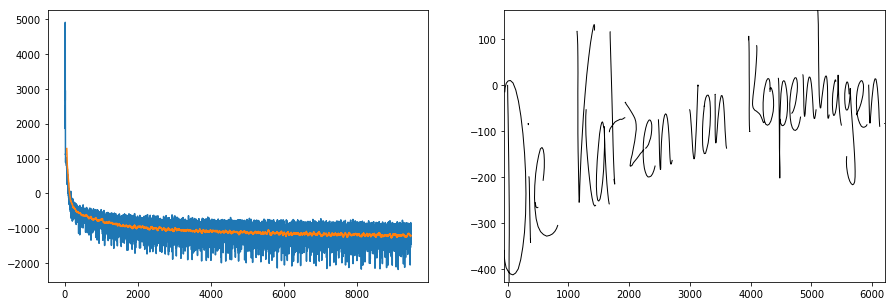time per minibatch 1.329148292541504
49 / 180 (of 208) : -1224.4349365234375
epoch 49 took 277.51844930648804 seconds


Sometimes I miss the output above, so here is the loss graph again.

In :
IPython.display.clear_output(wait=True)
pyplot.plot(numpy.fmin(losses,3000))
if len(losses)>=50:
smoothed = (numpy.convolve(losses,numpy.ones(50)/50,'valid'))
pyplot.plot(numpy.arange(len(losses)-len(smoothed),len(losses)),numpy.fmin(smoothed,3000))
print ("smoothloss", smoothed[-1])
pyplot.show()
print (epoch,'/', batch,'(of 208) :', res.item())

smoothloss -1229.02942749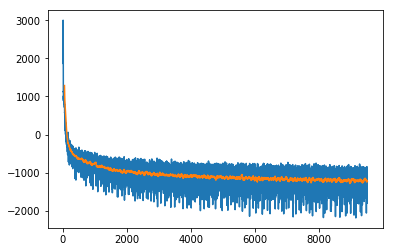49 / 189 (of 208) : -1108.754150390625


We can evaluate the negative log likelihood on the validation set. We should reach the same ballpark as Graves, who reports -1096.9 for the non-adaptive weight noise, albeit for a three-layer network) in Table 4.

In :
with torch.no_grad():
totalweight = 0.0
totalweightedloss = 0.0
for i in tqdm.tqdm(val_it): #, total=len(val_it)):
i_seq_pt = torch.stack([i.xs, i.ys, i.pen.float()], dim=2)
i_seq_tg = i_seq_pt[1:]
i_seq_pt = i_seq_pt[:-1]
i_seq_mask = (i.pen[:-1]>=0).float()
i_seq_str = i.txtn
i_seq_str_mask = i.txtmask
loss = m(i_seq_pt, i_seq_mask, i_seq_tg, i_seq_str, i_seq_str_mask).item()
lossweight = i.batch_size/val_it.batch_size
totalweight += lossweight
totalweightedloss += loss*lossweight
print("Negative log likelihood on validation set", totalweightedloss/totalweight)

100%|██████████| 25/25 [00:18<00:00,  1.37it/s]
Negative log likelihood on validation set -1123.9588082752182


If you want, you can load and save the model with the code below. It is more customary to use torch.save, but sometimes I like to save the weights only.

In :
import h5py
def save_to_hdf5(fn, d):
f = h5py.File(fn, "w")
for k,v in d.items():
f.create_dataset(k, data=v)
def load_from_hdf5(fn):
f = h5py.File(fn, "r")
print (list(f.keys()))
return {k:v[:] for k,v in f.items()}

if 0:
save_to_hdf5("graves_handwriting_generation_"+time.strftime("%Y-%m-%d-%H-%M",time.localtime())+"epoch_{}".format(epoch)+".hd5", {n:p.data.cpu().numpy() for n,p in m.named_parameters()})
if 0:
d =load_from_hdf5("graves_handwriting_generation_2018-03-13-02-45epoch_49.hd5")
for n,p in m.named_parameters():
p.data.copy_(torch.from_numpy(d[n]))


## Visual model evaluation¶

Nw that we have a model, we may show some samples. First we use the bias parameter as Graves describes in Section 5.4 and shows in Figure 16.

In :
for bias in [0,2,2,2,5,5,5,100,100,100]:
with torch.no_grad():
i = next(iter(train_it))
i_seq_pt = torch.stack([i.xs, i.ys, i.pen.float()], dim=2)
i_seq_pt = i_seq_pt[:-1]

i_seq_str = torch.autograd.Variable(torch.LongTensor([int(char_dict[c]) for c in "Die Katzen von Kopenhagen. "]).view(-1,1).cuda())
i_seq_str_mask = torch.autograd.Variable(torch.ones(i_seq_str.size()).cuda())
seq_pt, seq_mask = m.predict(i_seq_pt[0,:1], i_seq_str, i_seq_str_mask, bias=bias)
lengths = seq_mask.sum(0).data.long()
idx = 0
pyplot.figure(figsize=(10,2))
show_stroke(seq_pt.data.cpu()[:lengths[idx],idx])
#''.join([inv_char_dict[c] for c in i_seq_str.data.cpu()[:,idx]])
pyplot.title("bias={}".format(bias))
pyplot.show()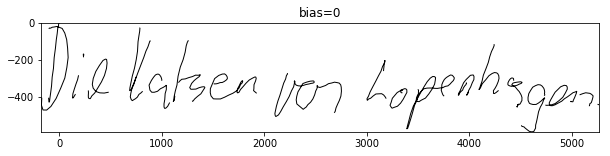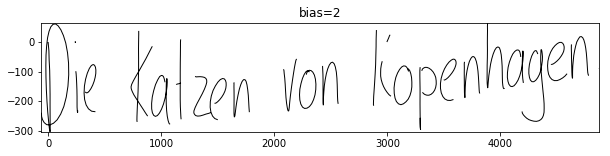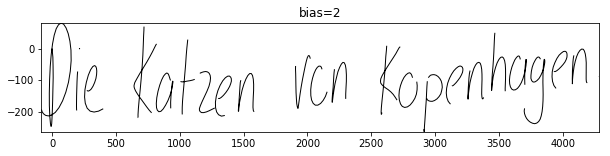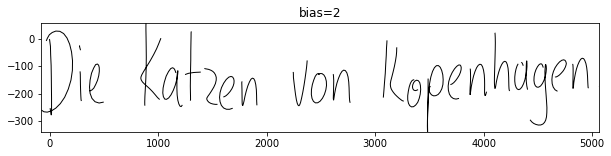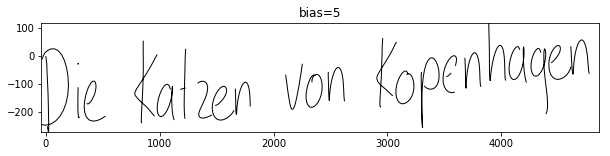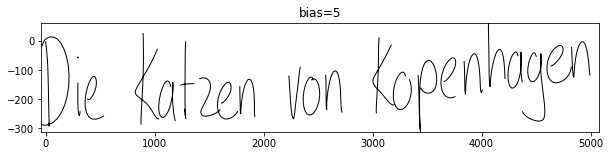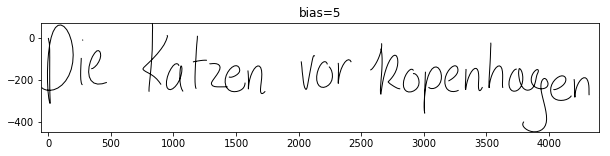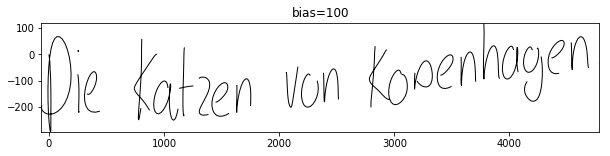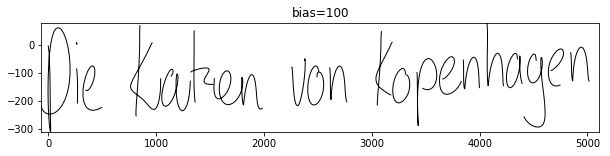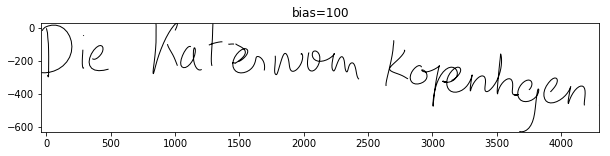Note that even with an extreme bias, the generated writing is not identical. This is in contrast to Graves' observation in Figure 16. I tentatively believe this is due to several modes sharing the top spot for the weights (e.g. two having mass almost exactly 0.5). Also the pen write vs. move probability is not biased.

### Model internal state¶

In Order to look at model internals, we can a pass a Python defaultdict to the model.

In :
d = collections.defaultdict(list)
with torch.no_grad():
i = next(iter(train_it))
i_seq_pt = torch.stack([i.xs, i.ys, i.pen.float()], dim=2)
i_seq_tg = i_seq_pt[1:]
i_seq_pt = i_seq_pt[:-1]
i_seq_mask = (i.pen[:-1]>=0).float()
i_seq_str = i.txtn
i_seq_str_mask = i.txtmask

opt.zero_grad()
res = m(i_seq_pt, i_seq_mask, i_seq_tg, i_seq_str, i_seq_str_mask, hidden_dict=d)
d.keys()

Out:
dict_keys(['seq_h', 'seq_k', 'seq_w', 'seq_phi', 'pi', 'mean_x', 'mean_y', 'std_x', 'std_y', 'rho', 'bernoulli', 'z', 'loss_per_timestep'])
In [ ]:


In :
idx=1
this_batch_size = i_seq_pt.size(1)
pts = i_seq_pt[:i.ptslen[0,idx],idx].cpu()
pts_len = i.ptslen[0,idx]
mean_y = d['mean_y'].view(-1,this_batch_size, d['mean_y'].size(1))[:pts_len,idx]*train_ds.coord_std+train_ds.coord_mean
mean_x = d['mean_x'].view(-1,this_batch_size, d['mean_x'].size(1))[:pts_len,idx]*train_ds.coord_std+train_ds.coord_mean
pi   = d['pi'].view(-1,this_batch_size, d['pi'].size(1))[:pts_len,idx]


The weights of the probabilities look reasonably similar to Graves Figure 14 in that we movements within strokes and in between being sampled from different mixture components.

In :
pyplot.figure(figsize=(15,5))
pyplot.imshow(pi.t(), aspect='auto')
#pts = torch.stack([i.xs,i.ys,i.pen.float()],dim=-1)
pyplot.figure(figsize=(15,5))
show_stroke(pts)
txt = train_ds.tensor_to_str(i.txt[:i.txtlen[0,idx],idx])
txt

Out:
'natural story-telling skill, it is'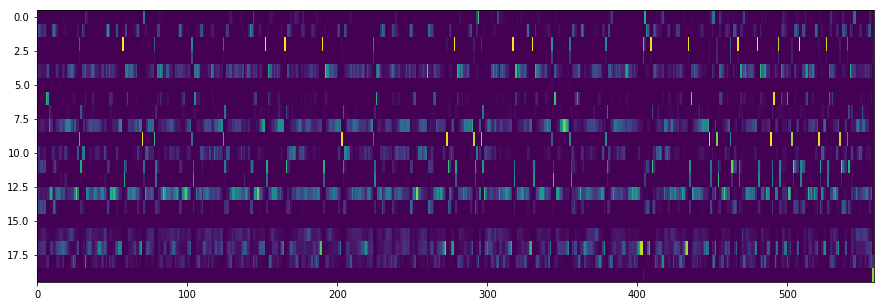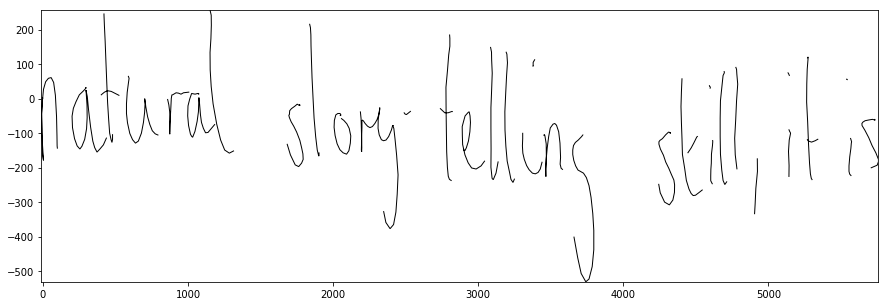Next we want to visualize the probability distributions for the points.

In :
def show_mixture_of_normals(x, y, sx, sy, rho, weights):
x = x.view(1,1,-1)
y = y.view(1,1,-1)
sx = sx.view(1,1,-1)
sy = sy.view(1,1,-1)
rho = rho.view(1,1,-1)
weights = weights.view(1,1,-1)
xmin, ymin = x.min(), y.min()
xmax, ymax = x.max(), y.max()
NX, NY = 1000, 70
xx = torch.arange(0., NX).view(1,-1,1)*(xmax-xmin)/NX+xmin
yy = torch.arange(0., NY).view(-1,1,1)*(ymax-ymin)/NY+ymin
x_s = (x-xx)/sx
y_s = (y-yy)/sy
tmp = 1-rho**2
log_dens = -(x_s**2+y_s**2-2*rho*x_s*y_s)/(2*tmp)-numpy.log(2*numpy.pi)-torch.log(sx)- torch.log(sy) - 0.5*torch.log(tmp)
dens = (weights*torch.exp(log_dens)).sum(-1)
pyplot.imshow(dens**0.25, aspect='auto') # note: the **0.25 is only for the color mapping

idx=1
this_batch_size = i_seq_pt.size(1)
pts = i_seq_pt[:i.ptslen[0,idx],idx].cpu()
pts_len = i.ptslen[0,idx]
cut_pts_len = min(pts_len, 100)
mean_x = d['mean_x'].view(-1,this_batch_size, d['mean_x'].size(1))[:cut_pts_len,idx]*train_ds.coord_std+train_ds.coord_mean
mean_y = d['mean_y'].view(-1,this_batch_size, d['mean_y'].size(1))[:cut_pts_len,idx]*train_ds.coord_std+train_ds.coord_mean
std_x  = d['std_x'].view(-1,this_batch_size,  d['std_x'].size(1))[:cut_pts_len,idx]*train_ds.coord_std
std_y  = d['std_y'].view(-1,this_batch_size,  d['std_y'].size(1))[:cut_pts_len,idx]*train_ds.coord_std
pi     = d['pi'].view(-1,this_batch_size,  d['pi'].size(1))[:cut_pts_len,idx].contiguous()
rho    = d['rho'].view(-1,this_batch_size, d['rho'].size(1))[:cut_pts_len,idx].contiguous()
_,maxidx = pi.max(1)
offset_x = torch.cat([torch.zeros(1),mean_x[torch.arange(0,len(mean_x), out=torch.LongTensor()), maxidx].cumsum(0)[:-1]],0)
offset_y = torch.cat([torch.zeros(1),mean_y[torch.arange(0,len(mean_y), out=torch.LongTensor()), maxidx].cumsum(0)[:-1]],0)

if 0:
NP = 10
x = torch.randn(NP)
y = torch.randn(NP)
sx = torch.exp(-1.5+0.5*torch.randn(NP))
sy = torch.exp(-1.5+0.5*torch.randn(NP))
rho = torch.zeros(NP)
weights = torch.ones(NP)
pyplot.figure(figsize=(15,5))
show_mixture_of_normals(mean_x+offset_x.unsqueeze(1), mean_y+offset_y.unsqueeze(1), std_x, std_y, rho, pi)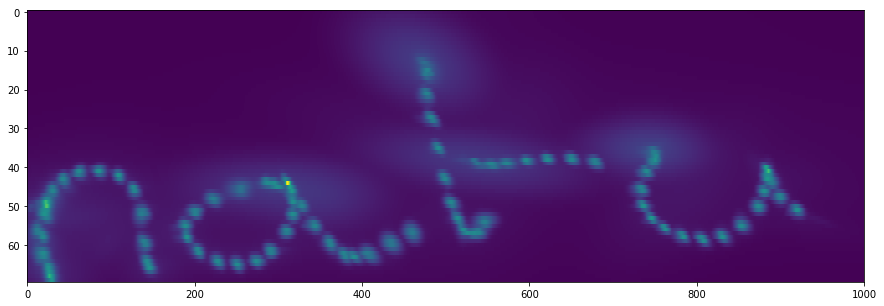In contrast to Figure 14 in Graves, we do not see that much of the increased uncertainty (but note with Graves that in Figure 14 the uncertainty is much smaller for the moves to a new stroke than in the non-conditioned output of Figure 10). This may mean our model is overfitting more than his. Graves uses regularisation with adaptive weighted noise which - to the best of my understanding - is very similar to the recently more popular Bayesian Variational Inference scheme.

We can also recreate figure 13. To illustrate which character is dominant in the attention mechanism, we color the strokes accordingly. (I first saw colored strokes at David Ha aka Hardmaru's blog, but at first glance he seems to color strokes, but we colorcode the attentention on chars.) It seems that the model looks at the next character quite early.

In :
seq_phi  = d['seq_phi'][:pts_len,:, idx]
pyplot.figure(figsize=(15,5))
pyplot.imshow(d['seq_phi'][:pts_len,:, idx].t(), aspect="auto")
ax = pyplot.gca()
ax.set_yticks(range(i.txtlen[0,idx].item()))
ax.set_yticklabels(list(txt))
pyplot.figure(figsize=(15,2))
colorlist = [pyplot.cm.jet(int(5*i/(seq_phi.size(1)-1)*(pyplot.cm.jet.N-1))%256) for i in range(seq_phi.size(1))]
colors = [colorlist[c] for c in seq_phi.max(1).tolist()]
show_stroke(pts, colors)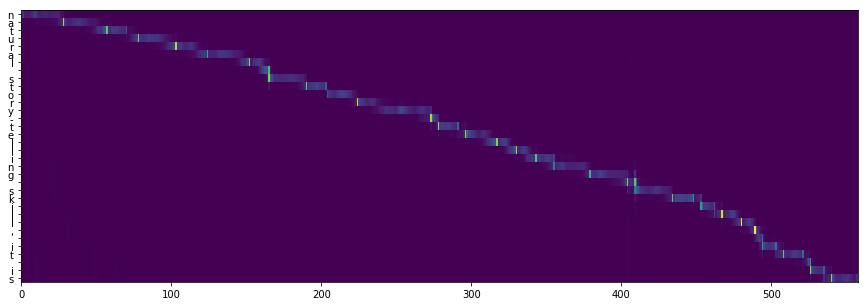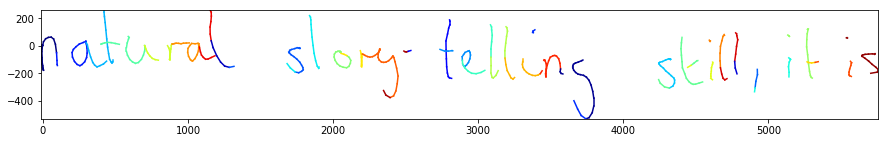I hope you enjoyed the exploration of Graves' classic model as much as I did writing it.

Feedback and corrections are always welcome at [email protected], I read and appreciate every mail.

Thomas Viehmann

In [ ]: